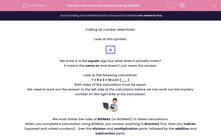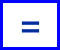# Solve the Calculations Using BIDMAS

In this worksheet, students will complete a calculation by adding in the missing number so that both sides are equal. They will need to follow the rules of BIDMAS. It will strengthen their number skills.This content is premium and exclusive to EdPlace subscribers.Key stage:  KS 2

Curriculum topic:   Verbal Reasoning

Curriculum subtopic:   Complete the Calculation

Difficulty level:#### Worksheet Overview

Calling all number detectives!

Look at this symbol:We know it is the equals sign but what does it actually mean?

It means the same as and doesn’t just mean the answer.

Look at the following calculation:

7 + 5 x 3 = 10 x 2+ ( __ )

Both sides of the calculation must be equal.

We need to work out the answer to the left side of the calculation before we can work out the mystery number on the right side of the calculation.We must follow the rules of BIDMAS (or BODMAS) in these calculations.

When you complete a calculation using BIDMAS, you answer anything in brackets first, then any indices (squared and cubed numbers),  then the division and multiplication parts, followed by the addition and subtraction parts.

Following the rules of BIDMAS, we must calculate 5 x 3 first and then add 7. This gives us 22.

So the answer on the right must equal 22. 10 x 2 is 20 so the mystery number must be 2 to make a total of 22.

7 + (5 x 3) = (10 x 2)+ 2

Remember to follow the rules of BIDMAS.

It’s now your turn to complete the calculation! Good luck!

### What is EdPlace?

We're your National Curriculum aligned online education content provider helping each child succeed in English, maths and science from year 1 to GCSE. With an EdPlace account you’ll be able to track and measure progress, helping each child achieve their best. We build confidence and attainment by personalising each child’s learning at a level that suits them.

Get started